## Abstract

This paper investigates the influence of thermophoresis on mixed convection heat and mass transfer flow over a vertical wavy surface in a porous medium with variable properties, namely variable viscosity and variable thermal conductivity. The effect of wavy surface is incorporated into non-dimensional equations by using suitable transformations and then transformed into non-linear ordinary differential equations by employing the similarity transformations and then solved numerically. The transport process of flow, heat and mass transfer in the boundary layer for aiding and opposing flow cases is discussed. The structure of flow, temperature and concentration fields in the Darcy porous media are more pronounced by complex interactions among variable viscosity, variable thermal conductivity, mixed convective parameter, thermophoresis and amplitude of the wavy surface. Increasing thermophoresis parameter enhances velocity profile, concentration distribution and Sherwood number while reduces Nusselt number. As increase in variable viscosity, temperature and concentration distributions are enhanced while velocity profile, Nusselt number and Sherwood numbers are reduced. This study finds applications in aerosol Technology, space technology and processes involving high temperatures.

## Keywords

Variable properties; Thermophoresis; Mixed convection; Vertical wavy surface; Darcy porous medium

## Nomenclature

${\textstyle {\overline {a}}}$- amplitude of the wavy surface

l- characteristic length of the wavy surface

${\textstyle {\overline {u}}}$- velocity component in the x-direction

${\textstyle {\overline {v}}}$- velocity component in the y-direction

Tw- wall temperature

T- ambient fluid temperature

Tr- reference temperature of the fluid

Cw- wall concentration

C- ambient fluid concentration

K- permeability of the porous medium

g- acceleration due to gravity

D- mass diffusivity

Ra- Darcy – Rayleigh number

Pe- Peclet number

N- buoyancy ratio

Le- Lewis number

Sc- Schmidt number

k- thermophoretic coefficient

Kn- Knudsen number

U- uniform free stream velocity

UT- thermophoretic velocity in x-direction

VT- thermophoretic velocity in y-direction

Nu- Nusselt number

Sh- Sherwood number

${\textstyle {\overline {x}}{\mbox{,}}{\overline {y}}}$- coordinate system

### Greek symbols

${\textstyle {\overline {\sigma }}}$- surface geometry function

μ- dynamic viscosity of the fluid

ν- kinematic viscosity of the fluid

ρ- fluid density

βt- coefficient of thermal expansion

βc- coefficient of mass expansion

α- thermal diffusivity

τ- thermophoresis parameter

${\textstyle {\overline {\psi }}}$- stream function

θ- non-dimensional temperature

${\textstyle \phi }$- non-dimensional concentration

Δ- mixed convective parameter

ξ- stream-wise coordinate

η- similarity variable (transverse coordinate)

### Subscripts

∞- conditions far away from the surface

T- caused by temperature

C- caused by concentration

w- at the wall

## 1. Introduction

Transport process in porous media can involve fluid, heat and mass transfer in single or multi-phase scenarios. Such flows with and without buoyancy effects arise frequently in many branches of engineering such as packed-bed or chemical catalytic reactors, nuclear waste repositories, and thermal insulation systems, drying of porous solids, underground energy transport and beds of fossil fuels. A good review of convective heat and mass transfer flows in a fluid saturated Darcy porous medium to date is given in Nield and Bejan .

Earlier studies on convective heat and mass transfer flow over a vertical plate in a porous medium have used Darcy law, which states volume averaged velocity is linear proportional to pressure gradient. This describes the transport process of momentum, thermal energy and concentration in fluid saturated porous media with low permeability properties such as sand, soil, human lung, lime stones and rocks. The interest in the study of convection flows due to the buoyancy effects caused by thermal and species variations in a fluid saturated porous medium over a vertical wavy surface has increased too many folds in recent years. This immense amount of interest has resulted into mired research articles addressing the wide range of problems involving the vertical wavy surface. Wavy surfaces are frequently encountered in many applications, for instance, utilizing in compact heat exchangers, cooling of electronic devices and systems and enhancing the heat and mass transfer efficiency of industrial transport process. The concept of convective boundary layer flow along a vertical wavy surface was first investigated by Yao  without the presence of porous medium and he  proposed a simple coordinate transformation to transform the effect of wavy surface on governing equations. Consequently, Rees and Pop ,  and  and Cheng  used this transformation and solved the governing boundary layer equations for free convection due to buoyancy driven flow along a vertical wavy surface in a porous media. They reported that the harmonic curves for local rate of heat and mass transfer coefficients have amplitude twice the amplitude of the wavy surface. Recently, Lakshminarayana and Sibanda  and Rathishkumar and Krishanmurthy  studied cross diffusion effects on natural convection from a vertical wavy surface in a Darcy and non-Darcy porous medium respectively. Neagu  analyzed double stratified effects on natural convective heat and mass transfer induced by a constant heat and mass fluxes vertical wall in a non-Darcy porous medium. Mahdy and Ahmed  examined free convective flow along a vertical wavy surface in a porous medium with a Nano-fluid. Siddiqa et al.  discussed surface radiation effects on free convective flow over a vertical wavy surface. Cheng  investigated natural convection boundary layer flow past a vertical sinusoidal surface in a bidisperse porous media. Hajmohammadi et al.  and  studied convection flow between two rotating cylinders filled with power law liquid and a microlayer of gas. Srinivasacharya et al. ,  and  studied thermal radiation and cross diffusion effects on convective heat and mass transfer flow over a vertical wavy surface in a porous medium with variable properties.

The above studies of thermal and solutal convection flow over a vertical wavy surface in a Darcy porous media have been analyzed based on constant fluid properties. However, it is well known that the fluid properties namely viscosity and thermal conductivity may vary with temperature. The enhancement of temperature causes to enhance transport process by decelerating viscosity across the thermodynamic boundary layer and due to which the rate of heat and mass transfer at the surface is more influenced. Nasser and Nader  studied variable properties on MHD unsteady double diffusive convective heat and mass transfer flow over a vertical wavy surface. Parveen and Alim  investigated the combined effects of variable properties and Joule heating on MHD natural convective heat and mass transfer flow over a vertical wavy surface. Alim et al.  considered and discussed conjugate effects of fluid properties on natural convective flow over a vertical wavy surface in the presence of heat generation. Hajmohammadi et al.  and Hajmohammadi and Nourazar  discussed conjugate analysis to study the effects of a thick plate and thermal conductivity on the excess temperature of iso-heat flux heat source cooled by forced convection flow. Bhuvanavijaya and Mallikarjuna  investigated variable thermal conductivity on convection boundary layer flow past a vertical plate in a rotating system with variable porosity regime. Srinivasacharya et al.  investigated variable viscosity and thermal conductivity effects on convection boundary layer flow along a sinusoidal surface in a porous medium with double dispersion effects. Srinivasacharya et al.  studied variable permeability on natural convection boundary flow over a vertical wavy surface in a porous medium.

The effect of thermophoresis encountered frequently in many fields, for instance aerosol technology, engineering and industrial applications, such as radioactive particle deposition in nuclear reactors, removal of particles from gas stream using filter beds, chemicals in acid rain can cause paint to peel, corrosion of steel structures such as bridges, erosion of stone statues and so on. In view of these applications the thermophoresis effect was analyzed in the following papers. Alam et al.  investigated the combined effect of thermophoresis and thermal radiation on MHD free-forced convective heat and mass transfer flow over a semi-infinite inclined porous plate with variable suction. Zueco et al.  used network simulation method to find numerical solutions of the governing equations pertaining to the MHD convective heat and mass transfer flow with lateral mass flux, heat source, Ohmic heating, thermal conductivity and thermophoresis. Puvi Arasu et al.  analyzed the thermophoretic effect on free convective heat and mass transfer under cross diffusion effects and variable stream conditions using Lie group analysis. Noor et al.  investigated the effect of thermophoresis on MHD convective heat and mass transfer flow over an inclined isothermal permeable surface with thermal radiation and heat source/sink. Rahman et al.  investigated thermophoretic particle deposition on unsteady convective flow over wedge with variable properties and variable Prandtl number with slip effects. Animasaun  discussed the thermophoretic, variable viscosity and thermal conductivity effects on convection boundary layer flow of non-Darcian MHD dissipative Casson fluid with suction and nth order of chemical reaction. Mallikarjuna et al.  investigated thermophoresis effects on nonlinear convection over a rotating cone in rotating fluid in a porous medium. Therefore, it is necessary to predict the flow, heat and mass transfer rates by taking into account temperature-dependent fluid properties.

To author’s best knowledge no one has investigated the influence of thermophoresis on mixed convective heat and mass transfer flow along a vertical wavy surface in a porous medium. The aim of the present study is to investigate combined effects of thermophoresis and variable properties on double diffusive mixed convection heat and mass transfer of a viscous fluid over a vertical wavy surface in a porous medium. The Darcy law is used to describe the transport process in fluid saturated porous medium with low permeability properties such as sand, soil, human lung, lime stones and rocks.

## 2. Formulation of the problem

We consider mixed convection in a fluid saturated Darcy porous medium with a Newtonian fluid bounded by vertical wavy surface with constant wall temperature Tw and concentration Cw. The temperature and concentration of the free stream region are T and C respectively. The flow is steady, laminar, and two dimensional. The configuration of the problem is shown in Fig. 1. The wavy surface is defined as

 ${\overline {\sigma }}({\overline {x}})={\overline {a}}sin\left({\frac {\pi {\overline {x}}}{l}}\right)$

where l   and ${\textstyle {\overline {a}}}$ are characteristic length and the amplitude of the wavy surface respectively. The wavy plate is considered along x-axis, x-coordinate is measured from its leading edge and y-coordinate is measured to the wavy plate. We assume that the porous medium is homogenous and isotropic and constant properties are local thermal equilibrium with fluid properties. All body forces are neglected. Assume that the fluid and the porous medium have constant physical properties except for the density variation required by the Boussinesq approximation. All properties are assumed independent of temperature except the viscosity and thermal conductivity. The temperature dependent viscosity and thermal conductivity are considered. In addition the thermophoresis effect is considered to discuss mass deposition variation on the wavy surface. In view of the above assumptions and Boussinesq approximation the governing equations for flow momentum, energy and concentration are:

 ${\frac {\partial {\overline {u}}}{\partial {\overline {x}}}}+$${\frac {\partial {\overline {v}}}{\partial {\overline {y}}}}=$$0$
(1)

 ${\frac {\partial }{\partial {\overline {y}}}}\left({\frac {\mu }{K}}{\overline {u}}\right)=$${\frac {\partial }{\partial {\overline {x}}}}\left({\frac {\mu }{K}}{\overline {v}}\right)\pm \rho g\left({\beta }_{t}{\frac {\partial T}{\partial {\overline {y}}}}+\right.$$\left.{\beta }_{c}{\frac {\partial C}{\partial {\overline {y}}}}\right)$
(2)

 ${\overline {u}}{\frac {\partial T}{\partial {\overline {x}}}}+$${\overline {v}}{\frac {\partial T}{\partial {\overline {y}}}}=$${\frac {\partial }{\partial {\overline {x}}}}\left(\alpha {\frac {\partial T}{\partial {\overline {x}}}}\right)+$${\frac {\partial }{\partial {\overline {y}}}}\left(\alpha {\frac {\partial T}{\partial {\overline {y}}}}\right)$
(3)

 ${\overline {u}}{\frac {\partial C}{\partial {\overline {x}}}}+$${\overline {v}}{\frac {\partial C}{\partial {\overline {y}}}}=$$D\left({\frac {{\partial }^{2}C}{\partial {\overline {x}}^{2}}}+\right.$$\left.{\frac {{\partial }^{2}C}{\partial {\overline {y}}^{2}}}\right)-$${\frac {\partial }{\partial {\overline {x}}}}(U_{T}C)-{\frac {\partial }{\partial {\overline {y}}}}(V_{T}C)$
(4)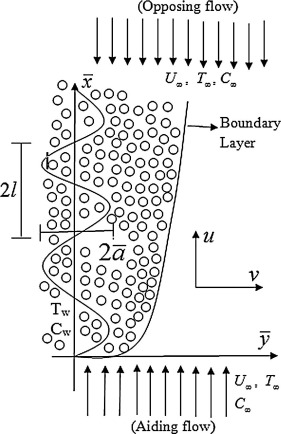Figure 1. Physical model and coordinate system.

The corresponding boundary conditions are

 ${\begin{array}{ll}{\overline {u}}=0{\mbox{,}}{\overline {v}}=0{\mbox{,}}T=T_{w}{\mbox{,}}C=C_{w}{\mbox{,}}&{\mbox{at}}\quad {\overline {y}}={\overline {\sigma }}({\overline {x}})={\overline {a}}sin\left({\frac {\pi {\overline {x}}}{l}}\right)\\{\overline {u}}\rightarrow U_{\infty {\mbox{,}}}T\rightarrow T_{\infty }{\mbox{,}}C\rightarrow C_{\infty }&{\mbox{as}}\quad {\overline {y}}\rightarrow \infty \end{array}}$
(5)

where ${\textstyle {\overline {u}}}$ and ${\textstyle {\overline {v}}}$ are the volume averaged velocity components in ${\textstyle {\overline {x}}}$ and ${\textstyle {\overline {y}}}$ directions, respectively. U is the uniform free stream velocity. In right hand side of Eq. (3), (+) sign represents the case when the buoyancy force is “aiding” the uniform free stream, (−) sign represents the buoyancy force is “opposing” the uniform free stream, T is temperature, C is the concentration, properties μ is the variable viscosity, ρ is the fluid density, K is the permeability of the porous medium, βt is the coefficients of thermal expansion, βc is the coefficient of concentration expansion, D is the mass diffusivity of the saturated porous medium and g is the acceleration due to gravity. The fluid properties are assumed to be isotropic and constant except the fluid viscosity and fluid thermal conductivity. The fluid viscosity is assumed to be varied as an inverse linear function of the temperature (see Lai and Kulacki ) as

 ${\frac {1}{\mu }}={\frac {1}{{\mu }_{\infty }}}(1+\delta (T-$$T_{\infty }))\quad {\mbox{or}}\quad {\frac {1}{\mu }}=b(T-$$T_{r})$
(6)

where ${\textstyle b={\frac {\delta }{{\mu }_{\infty }}}}$ and ${\textstyle T_{r}=T_{\infty }-{\frac {1}{\delta }}}$. Both b and Tr are constants and their values depend on the reference state and the thermal property of the fluid i.e. δ. θr  , which is defined by ${\textstyle {\theta }_{r}={\frac {T_{r}-T_{\infty }}{T_{w}-T_{\infty }}}=}$$-{\frac {1}{\delta (T_{w}-T_{\infty })}}$ is constant. It is important to note that for δ → 0 (i.e. μ = μ = constant) then ${\textstyle {\theta }_{r}\rightarrow \infty }$. It is also mentioning here that b > 0 for liquids and b < 0 for gases. Thus, ${\textstyle {\theta }_{r}}$ is positive for gases and negative for liquids. T is the free stream temperature. Also, we assume that the fluid thermal conductivity α is assumed to vary as a linear function of temperature in the form of (see Seddeek and Salem )

 $\alpha ={\alpha }_{o}(1+E(T-T_{\infty }))$

where α0 is the thermal diffusivity at the wavy surface temperature Tw and E is a constant depending on the nature of the fluid. It is worth mentioning here that E < 0 for fluids such as lubrication oils, while E > 0 for fluids such as air and water. This can be written in the non-dimensional form (see Slattery ) as

 $\alpha ={\alpha }_{o}(1+\beta \theta )$
(7)

where ${\textstyle \beta =E(T_{w}-T_{\infty })}$ is the thermal conductivity parameter and Tw is the wavy surface temperature.

In Eq. UT and VT are thermophoretic velocities which can be written as (see Wu and Greif )

 $U_{T}=-{\frac {k\nu }{T_{r}}}{\frac {\partial T}{\partial {\overline {x}}}}\quad {\mbox{and}}\quad V_{T}=$$-{\frac {k\nu }{T_{r}}}{\frac {\partial T}{\partial {\overline {y}}}}$
(8)

where k is thermophoretic coefficient which ranges in the values between 0.2 and 1.2 and is defined as (see Talbot et al. )

 $k={\frac {2C_{s}({\lambda }_{g}/{\lambda }_{p}+C_{t}Kn)\left[1+Kn(C_{1}+C_{2}e^{-C_{3}/Kn})\right]}{\left(1+3C_{m}Kn)(1+2{\lambda }_{g}/{\lambda }_{p}+2C_{t}Kn\right)}}$

where C1, C2, C3, Cm, Cs, and Ct are constants, λg and λp are thermal conductivities of fluid and diffused particles, respectively and Kn is the Knudsen number.

We define the stream function ${\textstyle {\overline {\psi }}}$, which should satisfy the continuity Eq. (1) such that

 ${\overline {u}}={\frac {\partial {\overline {\psi }}}{\partial {\overline {y}}}}{\mbox{,}}\quad {\overline {v}}=$$-{\frac {\partial {\overline {\psi }}}{\partial {\overline {x}}}}$

In order to write the governing boundary layer equations in non-dimensional form, we introduce the following dimensionless variables:

 ${\begin{array}{lll}x={\overline {x}}/l{\mbox{,}}&y={\overline {y}}/l{\mbox{,}}\quad a={\overline {a}}/l{\mbox{,}}&\sigma ={\overline {\sigma }}/l\\{\psi }^{_{\ast }}={\overline {\psi }}/{\alpha }_{o}{\mbox{,}}&\theta ={\frac {T-T_{\infty }}{T_{w}-T_{\infty }}}{\mbox{,}}&\phi ={\frac {C-C_{\infty }}{C_{w}-C_{\infty }}}\end{array}}$
(9)

Using Eqs. (6), (7), (8) and (9), Eqs. (2), (3) and (4) become

 ${\frac {1}{\theta -{\theta }_{r}}}\left({\frac {\partial \theta }{\partial y}}{\frac {\partial {\psi }^{_{\ast }}}{\partial y}}+\right.$$\left.{\frac {\partial \theta }{\partial x}}{\frac {\partial {\psi }^{_{\ast }}}{\partial x}}\right)+$${\frac {{\partial }^{2}{\psi }^{_{\ast }}}{\partial y^{2}}}+$${\frac {{\partial }^{2}{\psi }^{_{\ast }}}{\partial x}}=$$\pm Ra\left(1-{\frac {\theta }{{\theta }_{r}}}\right)\left({\frac {\partial \theta }{\partial y}}+\right.$$\left.N{\frac {\partial \phi }{\partial y}}\right)$
(10)

 ${\frac {\partial {\psi }^{_{\ast }}}{\partial y}}{\frac {\partial \theta }{\partial x}}-$${\frac {\partial {\psi }^{_{\ast }}}{\partial x}}{\frac {\partial \theta }{\partial y}}=$$\beta \left({\left({\frac {\partial \theta }{\partial x}}\right)}^{2}+\right.$$\left.{\left({\frac {\partial \theta }{\partial y}}\right)}^{2}\right)+$$(1+\beta \theta )\left({\frac {{\partial }^{2}\theta }{\partial x^{2}}}+\right.$$\left.{\frac {{\partial }^{2}\theta }{\partial y^{2}}}\right)$
(11)

 $Le\left({\frac {\partial {\psi }^{_{\ast }}}{\partial y}}{\frac {\partial \phi }{\partial x}}-\right.$$\left.{\frac {\partial {\psi }^{_{\ast }}}{\partial x}}{\frac {\partial \phi }{\partial y}}\right)=$${\frac {{\partial }^{2}\phi }{\partial x^{2}}}+{\frac {{\partial }^{2}\phi }{\partial y^{2}}}-$$Sc\tau \left({\frac {{\partial }^{2}\theta }{\partial x^{2}}}\phi +\right.$$\left.{\frac {{\partial }^{2}\theta }{\partial y^{2}}}\phi +\right.$$\left.{\frac {\partial \theta }{\partial x}}{\frac {\partial \phi }{\partial x}}+\right.$$\left.{\frac {\partial \theta }{\partial y}}{\frac {\partial \phi }{\partial y}}\right)$
(12)

where ${\textstyle Ra={\frac {g{\beta }_{t}K(T_{w}-T_{\infty })l}{{\alpha }_{o}\nu }}}$ is the Darcy–Rayleigh number, ${\textstyle \nu ={\frac {{\mu }_{\infty }}{\rho }}}$ is the kinematic viscosity of the fluid, ${\textstyle N={\frac {{\beta }_{c}(C_{w}-C_{\infty })}{{\beta }_{t}(T_{w}-T_{\infty })}}}$ is the buoyancy ratio, ${\textstyle Le={\frac {{\alpha }_{o}}{D}}}$ is the Lewis number, ${\textstyle \tau =-{\frac {k}{T_{r}}}(T_{w}-T_{\infty })}$ is the Thermophoretic parameter, and ${\textstyle Sc={\frac {\nu }{D}}}$ is the Schmidt number.

The associated boundary conditions are given by

 ${\begin{array}{lllll}{\psi }^{_{\ast }}=0{\mbox{,}}&\theta =1{\mbox{,}}&\phi =1{\mbox{,}}&{\mbox{on}}&y=asin(x){\mbox{,}}\\{\psi }_{y}^{_{\ast }}\rightarrow {\frac {{\alpha }_{0}}{l}}U_{\infty }{\mbox{,}}&\theta \rightarrow 0&{\mbox{as}}&y\rightarrow \infty {\mbox{,}}\end{array}}$
(13)

Let us consider the following transformations:

 $x=\xi {\mbox{,}}\quad {\hat {\eta }}={\frac {y-asin(x)}{{\xi }^{1/2}{Pe}^{-1/2}}}{\mbox{,}}\quad {\psi }^{_{\ast }}=$${Pe}^{1/2}\psi {\mbox{.}}$
(14)

Substituting Eq. (14) into Eqs. (10), (11), (12) and (13) and letting Pe → ∞, to transfer the effect of the wavy surface from the boundary conditions into governing equations, we obtain the following boundary layer equations:

 ${\frac {1}{\theta -{\theta }_{r}}}(1+a^{2}{cos}^{2}\xi ){\frac {\partial \theta }{\partial {\hat {\eta }}}}{\frac {\partial \psi }{\partial {\hat {\eta }}}}+$$(1+a^{2}{cos}^{2}\xi ){\frac {{\partial }^{2}\psi }{\partial {\hat {\eta }}^{2}}}=$$\pm \Delta {\xi }^{1/2}\left(1-{\frac {\theta }{{\theta }_{r}}}\right)\left({\frac {\partial \theta }{\partial {\hat {\eta }}}}+\right.$$\left.N{\frac {\partial \psi }{\partial {\hat {\eta }}}}\right)$
(15)

 $\beta (1+a^{2}{cos}^{2}\xi ){\left({\frac {\partial \theta }{\partial {\hat {\eta }}}}\right)}^{2}+$$(1+\beta \theta )(1+a^{2}{cos}^{2}\xi ){\frac {{\partial }^{2}\theta }{\partial {\hat {\eta }}^{2}}}=$${\xi }^{1/2}\left({\frac {\partial \psi }{\partial {\hat {\eta }}}}{\frac {\partial \theta }{\partial \xi }}-\right.$$\left.{\frac {\partial \psi }{\partial \xi }}{\frac {\partial \theta }{\partial {\hat {\eta }}}}\right)$
(16)

 $(1+a^{2}{cos}^{2}\xi ){\frac {{\partial }^{2}\phi }{\partial {\hat {\eta }}^{2}}}-$$Sc\tau \left((1+a^{2}{cos}^{2}\xi )\phi {\frac {{\partial }^{2}\theta }{\partial {\hat {\eta }}^{2}}}+\right.$$\left.(1+a^{2}{cos}^{2}\xi ){\frac {\partial \theta }{\partial {\hat {\eta }}}}{\frac {\partial \phi }{\partial {\hat {\eta }}}}\right)=$$Le{\xi }^{1/2}\left({\frac {\partial \psi }{\partial {\hat {\eta }}}}{\frac {\partial \phi }{\partial \xi }}-\right.$$\left.{\frac {\partial \psi }{\partial \xi }}{\frac {\partial \phi }{\partial {\hat {\eta }}}}\right)$
(17)

In order to transform Eqs. (15), (16) and (17) into a set of ordinary differential equations, we introduce the following similarity transformations:

 $\eta ={\frac {\hat {\eta }}{1+a^{2}{cos}^{2}\xi }}{\mbox{,}}\quad \psi =$${\xi }^{1/2}f(\eta ){\mbox{,}}\quad \theta =\theta (\eta )\quad {\mbox{and}}\quad \phi =$$\phi ({\hat {\eta }})$
(18)

Thus, we obtain

 $f^{''}+{\frac {1}{\theta -{\theta }_{r}}}{\theta }^{'}f^{'}=$$\pm \Delta \left(1-{\frac {\theta }{{\theta }_{r}}}\right)({\theta }^{'}+$$N{\phi }^{'})$
(19)

 $\beta {\left({\theta }^{'}\right)}^{2}+(1+\beta \theta ){\theta }^{''}+$${\frac {1}{2}}f{\theta }^{'}=0$
(20)

 ${\phi }^{''}+\left({\frac {1}{2}}Lef-Sc\tau {\theta }^{'}\right){\phi }^{'}-$$Sc\tau \phi {\theta }^{''}=0$
(21)

where prime denotes differentiation with respect to ${\textstyle \eta }$.

The associated boundary conditions are

 $f=0{\mbox{,}}\quad \theta =1{\mbox{,}}\quad {\mbox{and}}\quad \phi =$$1\quad {\mbox{at}}\quad \eta =0$ $f^{'}\rightarrow 1{\mbox{,}}\quad \theta \rightarrow 0\quad {\mbox{and}}\quad \phi \rightarrow 0\quad {\mbox{as}}\quad \eta \rightarrow \infty$
(22)

In Eq. (19)N represents buoyancy ratio, i.e., ratio of thermal buoyancy to the solutal buoyancy forces. If N = 0, the flow is induced by only thermal buoyancy force. Negative and positive values of N take opposing buoyancy and aiding buoyancy flow effects, respectively. In the same Eq. (19), ${\textstyle \Delta ={\frac {Ra}{Pe}}}$ represents mixed convective parameter. Mixed convective flow is the combination of free convection, caused by buoyancy force, and forced convection, due to the external force. It is noted that when Pe → ∞, the flow is induced by free convection and when Pe → 0, the flow is induced by purely forced convection. If Δ = 1, the flow is induced by both free and forced convective flows. In Eq. (19), ${\textstyle {\theta }_{r}}$ represents variable viscosity parameter, which is defined as ${\textstyle {\theta }_{r}={\frac {T_{r}-T_{\infty }}{T_{w}-T_{\infty }}}=}$$-{\frac {1}{\delta (T_{w}-T_{\infty })}}$. Moreover, we say that for heated surfaces, i.e., ${\textstyle T_{w}-T_{\infty }>0}$, ${\textstyle {\theta }_{r}}$ must be greater than unity for gases and less than zero for liquids. But for cold surfaces ${\textstyle \left(T_{w}-T_{\infty }<0\right)}$, ${\textstyle {\theta }_{r}}$ gives opposite results, i.e., ${\textstyle {\theta }_{r}}$ must be greater than unity for liquids and less than zero for gases. It is important to note that when δ → 0 gives rise to θr → ∞, and in this case Eq. (19) reduces to that of constant viscosity. Similarly when the variable thermal conductivity parameter ${\textstyle \beta \rightarrow 0}$, Eq. (20) reduces to that of constant thermal conductivity. In Eq. (21), ${\textstyle \tau =-{\frac {k}{T_{r}}}(T_{w}-T_{\infty })}$ represents thermophoresis parameter. From the practical point of view two possible cases exist, (i) heated surfaces ${\textstyle \left(T_{w}-T_{\infty }>0\right)}$, which leads to ${\textstyle \tau <0}$, (ii) cold surfaces ${\textstyle \left(T_{w}-T_{\infty }<0\right)}$, which gives rise to ${\textstyle \tau >0}$. For the second case thermophoresis is the special type of mechanism for capture of particles on cold surfaces, being especially important for submicron particles since thermophoretic velocity is relatively independent of particle size, while the first one may be thought of suppressing the particle deposition on the surface. It concludes that for heated surfaces a dust-free area is obtained adjacent to the surface due to particles moving away from the surface; thus, fluid particle concentration is tending to zero near the surface. Hence, thermophoretic velocity is treated as particle deposition velocity when ${\textstyle \tau <0}$.

The main results of practical interest in many applications are heat and mass transfer coefficient at the surface. The heat and mass transfer coefficients are expressed in terms of Nusselt number ${\textstyle {Nu}_{x}}$ and Sherwood number ${\textstyle {Sh}_{x}}$. Nusselt number is given by

 ${Nu}_{x}={\frac {{xq}_{w}}{{\alpha }_{0}(T_{w}-T_{\infty })}}$
(23)

where qw   is the heat flux on the wavy surface and is defined by ${\textstyle q_{w}=-{\alpha }_{0}{\overline {n}}\cdot \nabla T}$, ${\textstyle {\overline {n}}=\left({\frac {-aCos(\xi )}{\sqrt {1+a^{2}{cos}^{2}(\xi )}}}{\mbox{,}}{\frac {1}{\sqrt {1+a^{2}{cos}^{2}(\xi )}}}\right)}$ is the unit normal vector to the wavy surface, and ${\textstyle {\alpha }_{o}}$ is the effective porous medium thermal conductivity.

In non-dimensional form

 ${Nu}_{\xi }={\frac {-{\theta }^{'}(0){Pe}_{\xi }^{1/2}}{{\left(1+a^{2}{cos}^{2}(\xi )\right)}^{1/2}}}$
(24)

Similarly we obtain the Sherwood number

 ${Sh}_{\xi }={\frac {-{\phi }^{'}(0){Pe}_{\xi }^{1/2}}{{\left(1+a^{2}{cos}^{2}(x)\right)}^{1/2}}}$
(25)

## 3. Results and discussions

The set of highly non-linear coupled Eqs. (19), (20) and (21) of second order in fθ and ϕ, subject to the boundary conditions (22) are solved by using efficient numerical method with shooting technique that uses fourth order Runge–Kutta method and Newton–Raphson method (Mallikarjuna et al. ). In order to check the effects of step size ${\textstyle \left(\Delta \eta \right)}$ we found the Nusselt number and Sherwood number with four different step sizes as ${\textstyle \Delta \eta =0.1{\mbox{,}}\quad \Delta \eta =0.01{\mbox{,}}\quad \Delta \eta =}$$0.001$ and ${\textstyle \Delta \eta =0.0001}$ .We observe from Table 1 that the results are independent with the step size ${\textstyle \left(\Delta \eta \right)}$. Hence a step size ${\textstyle \Delta \eta =0.01}$ is selected to be satisfactory for a convergence criterion of 10−6 in all cases. In order to verify the accuracy of the present method, we compared the Nusselt number and Sherwood results with those of previous existing results reported by Chin et al.  in the absence of thermophoresis parameter for vertical flat plate (i.e., a = 0) and Lai  in the absence of variable properties and thermophoresis parameter for vertical flat plate (i.e., a = 0). The comparison results were found to be in a very good agreement with Chin et al.  and Lai  as shown in Table 2 and Table 3.

Table 1. Grid-independence for β = 0.5, a = 0.5, τ = 1, N = 1, Sc = 0.6, Le = 0.5, Δ = 1 and θr = 3.
Δη (Step size) ${\textstyle {Nu}_{\xi }{Ra}_{\xi }^{-1/2}}$ ${\textstyle {Sh}_{\xi }{Ra}_{\xi }^{-1/2}}$
0.1 0.4673888944 0.3812042945
0.01 0.4673886097 0.3812047348
0.001 0.4673883736 0.3812042401
0.0001 0.4673885689 0.3812046549

Table 2. Comparison of Nusselt number results for a = 0, β = 0, τ = 0 and N = 0.
Parameter ${\textstyle {Nu}_{\xi }{Pe}_{\xi }^{-1/2}}$ (Δ = 1) ${\textstyle {Nu}_{\xi }{Pe}_{\xi }^{-1/2}}$ (Δ = 10)
θr Chin et al.  Present Chin et al.  Present
1 0.8603 −0.5640 1.2908 −1.6344
2 0.9435 −1.1058 1.7748 −1.9600
4 0.9821 −1.3860 1.9689 −2.3672

Table 3. Comparison of Nusselt number and Sherwood number for a = 0, θr = 0, β = 0 and τ = 0 at N = 0.5, Le = 1.
Parameter ${\textstyle {Nu}_{\xi }{Pe}_{\xi }^{-1/2}}$ ${\textstyle {Sh}_{\xi }{Pe}_{\xi }^{-1/2}}$
Δ Lai  Present Lai  Present
0.1 −0.5640 −0.5640 −1.6344 −1.6344
1.0 −1.1060 −1.1058 −1.9599 −1.9600
1.5 −1.3860 −1.3860 −2.3674 −2.3672

Numerical results for the flow field variables velocity, temperature and concentration as well as the coefficient of rate of heat and mass transfer have been obtained for various values of variable viscosity parameter, variable thermal conductivity parameter, thermophoresis parameter, mixed convection parameter and the amplitude of the wavy surface. Figure 2, Figure 3 and Figure 4 represent the variation of variable viscosity parameter (θr) on velocity (u), temperature (θ), and concentration (ϕ  ) distributions respectively. It is noticed from these figures that increasing variable viscosity parameter retards the flow velocity while enhancing temperature and concentration profile for both cases of aiding flow and opposing flow cases. Thus, the hydrodynamic velocity boundary layer thickness decreases with increase in ${\textstyle {\theta }_{r}}$ but increases in thermal and solutal boundary layer thickness.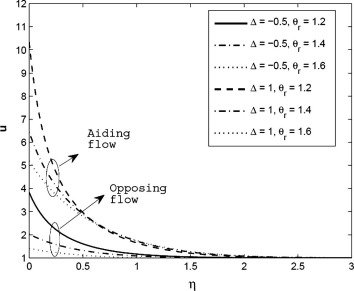Figure 2. Velocity profile for different values of variable viscosity parameter (θr) for β = 0.5, N = 1, Le = 1, Sc = 0.6, τ = 1.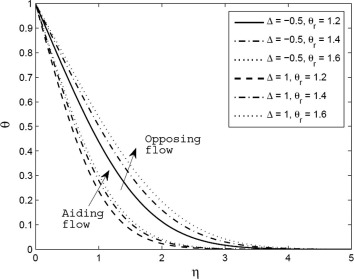Figure 3. Temperature profile for different values of variable viscosity parameter (θr) for β = 0.5, N = 1, Le = 1, Sc = 0.6, τ = 1.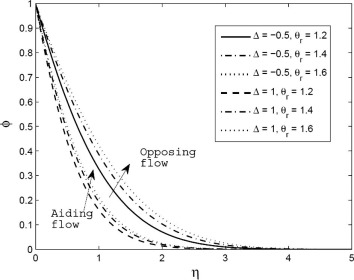Figure 4. Concentration profile for different values of variable viscosity parameter (θr) for β = 0.5, N = 1, Le = 1, Sc = 0.6, τ = 1.

Figure 5, Figure 6 and Figure 7 depict the velocity, temperature and concentration distributions for different values of variable thermal conductivity parameter (β) respectively. It is observed that velocity and temperature distributions are more pronounced with increase in β and hence these results are considerably increased within the boundary layer region for both cases of aiding and opposing flow. Conversely, the concentration profiles decreased significantly with increase in β for both cases of aiding and opposing flow.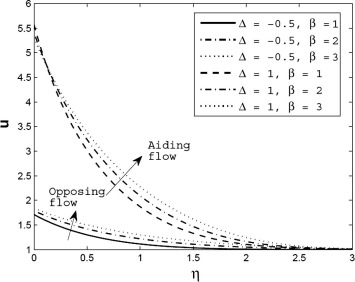Figure 5. Velocity profile for different values of variable thermal conductivity parameter (β) for θr = 1.5, N = 1, Le = 1, Sc = 0.6, τ = 1.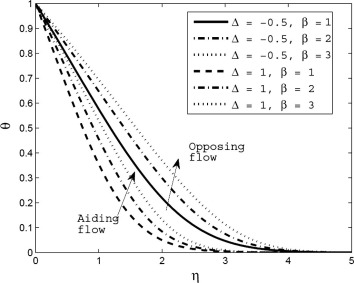Figure 6. Temperature profile for different values of variable thermal conductivity parameter (β) for θr = 1.5, N = 1, Le = 1, Sc = 0.6, τ = 1.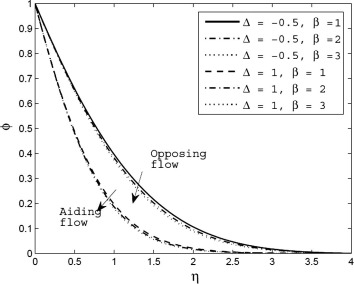Figure 7. Concentration profile for different values of variable thermal conductivity parameter (β) for θr = 1.5, N = 1, Le = 1, Sc = 0.6, τ = 1.

Figure 8, Figure 9 and Figure 10 show velocity, temperature and concentration distributions for different values of thermophoresis parameter (τ) respectively. Increase in τ tends to increase the flow velocity for opposing flow case but these results are reversed for aiding flow case. Conversely, temperature profile is reversed, compared to velocity profile. That is increasing τ results in an enhancement in temperature profile for aiding flow case while reducing the temperature profile for opposing flow case. The solutal boundary layer thickness reduces with increase in τ for both cases of aiding and opposing flow.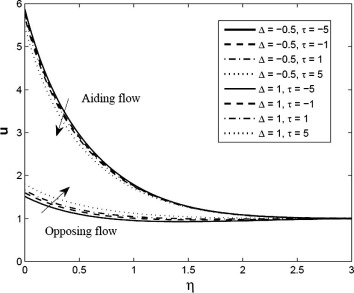Figure 8. Velocity profile for different values of thermophoresis parameter (τ) for θr = 1.5, β = 0.5, N = 1, Le = 1, Sc = 0.6.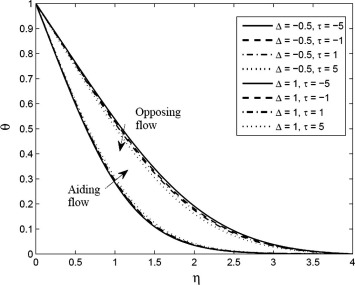Figure 9. Temperature profile for different values of thermophoresis parameter (τ) for θr = 1.5, β = 0.5, N = 1, Le = 1, Sc = 0.6.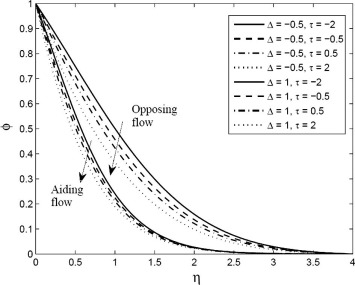Figure 10. Concentration profile for different values of thermophoresis parameter (τ) for θr = 1.5, β = 0.5, N = 1, Le = 1, Sc = 0.6.

Figure 11 and Figure 12 represent the axial distributions of Nusselt number and Sherwood number for different values of ${\textstyle {\theta }_{r}}$ respectively. We observed from these figures that the results of Nusselt number and Sherwood number are decreased with increase in ${\textstyle {\theta }_{r}}$ i.e. amplitude of Nusselt number and Sherwood number is reduced for higher values of ${\textstyle {\theta }_{r}}$ for both cases of aiding and opposing flow. Fig. 13 shows that increase in β causes to decrease the amplitude of the Nusselt number for both aiding and opposing flow cases. As increase in β, Sherwood number decreases for aiding flow case but the opposite results are noted for opposing flow case as given Fig. 14. Figure 15 and Figure 16 illustrate the variation of τ on Nusselt number and Sherwood number with stream wise coordinate (ξ) respectively. From Fig. 15 we noticed that increase in τ tends to decrease the Nusselt number considerably for aiding flow case but the opposite trend is observed for opposing flow case. Sherwood number results are increased more significantly with increase in τ for both cases of aiding and opposing flow. Figure 17 and Figure 18 depict the variation of the amplitude of the wavy surface (a) on Nusselt number and Sherwood number respectively. An increase in the amplitude of the wavy surface (a) shows depreciation on the amplitude of the Nusselt number and Sherwood number for both cases of aiding and opposing flow. It is important to note that for a = 0 the waviness is negligible, i.e. the wavy surface becomes the flat surface.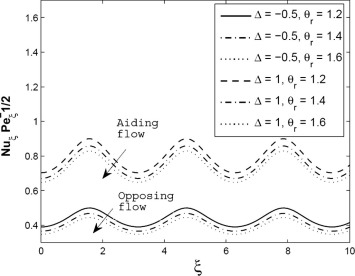Figure 11. Axial distributions of Nusselt number for different values of variable viscosity parameter (θr) for β = 0.5, N = 1, Le = 1, τ = 1, a = 0.5.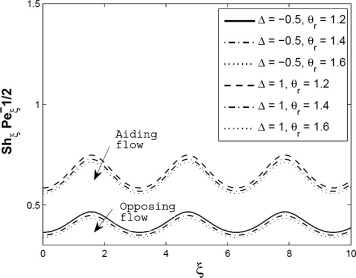Figure 12. Axial distributions of Sherwood number for different values of variable viscosity parameter (θr) for β = 0.5, N = 1, Le = 1, τ = 1, a = 0.5.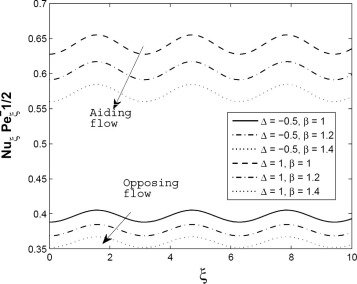Figure 13. Axial distributions of Nusselt number for different values of variable thermal conductivity parameter (β) for θr = 1.5, N = 1, Le = 1, τ = 1, a = 0.5.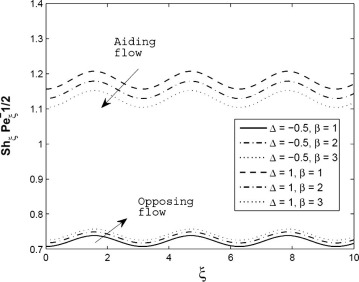Figure 14. Axial distributions of Sherwood number for different values of variable thermal conductivity parameter (β) for θr = 1.5, N = 1, Le = 1, τ = 1, a = 0.5.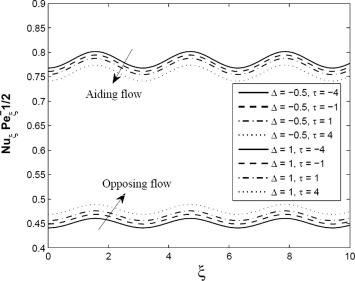Figure 15. Axial distributions of Nusselt number for different values of thermophoresis parameter (τ) for θr = 1.5, β = 0.5, N = 1, Le = 1, a = 0.5.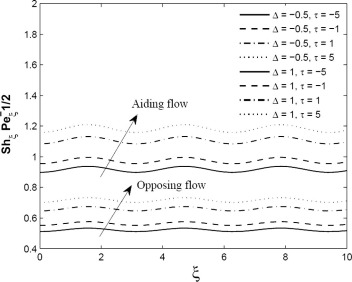Figure 16. Axial distributions of Sherwood number for different values of thermophoresis parameter (τ) for θr = 1.5, β = 0.5, N = 1, Le = 1, a = 0.5.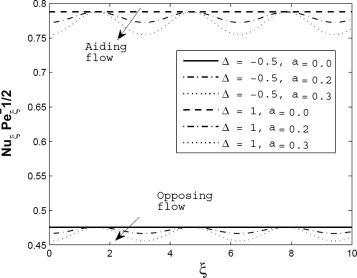Figure 17. Axial distributions of Nusselt number for different values of the amplitude of the wavy surface (a) for θr = 1.5, β = 0.5, N = 1, Le = 1, τ = 1.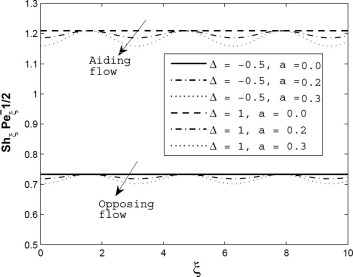Figure 18. Axial distributions of Sherwood number for different values of the amplitude of the wavy surface (a) for θr = 1.5, β = 0.5, N = 1, Le = 1, τ = 1.

It is important to notice that heat and mass characteristics are larger for the negative values of mixed convective parameter (i.e., opposing flow case) while flow field variable is larger for positive values of mixed convective parameter (i.e., aiding flow case) with increasing values of various physical parameters. We also conclude that the amplitude of the rate of heat and mass transfer is influenced considerably and is larger for opposing flow case, in comparison with aiding flow for various values of physical parameters.

## 4. Conclusions

• Increase in variable viscosity results in an enhancement in temperature and concentration distributions while depreciation in velocity profile and Nusselt number and Sherwood number.
• Velocity and temperature distributions are increased but concentration profile and Nusselt number results are decreased with increase in variable thermal conductivity for both cases of aiding and opposing flows. The Sherwood number increases for opposing flow case and reduces for aiding flow case for larger values of variable thermal conductivity.
• Increase in thermophoresis parameter causes to reduce concentration profile while enhancing the Sherwood number results considerably for both cases of aiding and opposing flows. Velocity and Nusselt number are significantly reduced but enhanced the temperature profile for aiding flow case but the opposite results are obtained for opposing flow cases as increase in thermophoresis parameter.
• For higher values of the amplitude of the wavy surface, the Nusselt number and Sherwood number results are reduced for both cases of aiding and opposing flows.
• It is noted that for aiding flow case Velocity profiles, Nusselt number and Sherwood number results are larger for aiding flow case but temperature and concentration profiles are smaller in comparison with opposing flow case.

## Acknowledgments

One of the authors Dr. B. Mallikarjuna wishes to thank the Department of Science and Technology, New Delhi, India, for providing financial support to enable conducting this research work under Inspire Fellowship Program and B.M.S. College of Engineering, Bangalore-19, for giving support to modify the paper through the TECHNICAL EDUCATION QUALITY IMPROVEMENT PROGRAMME [TEQIP-II] of the MHRD, Government of India.

### Document informationPublished on 12/04/17

Licence: Other

### Document Score0

Views 16
Recommendations 0